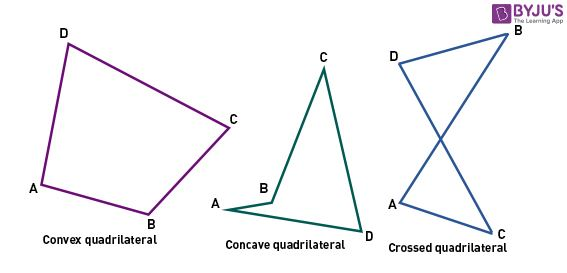A quadrilateral is a plane figure consisting of four sides and four angles. Sometimes, these are also called tetragons or quadrangles. Quadrilaterals can be classified into several types, such as regular, irregular, convex, concave, crossed, etc. A simple quadrilateral is a quadrilateral that is not intersecting. However, concave and Convex quadrilaterals are also considered as simple quadrilaterals.

In convex quadrilaterals, both diagonals lie in the quadrilateral, and all the interior angles are less than 180 degrees. In the case of the concave quadrilateral, one of the two diagonals lies outside the quadrilateral, and one interior angle is greater than 180 degrees. There are different math formulas to solve questions based on these types of quadrilaterals.Quadrilateral Worksheets are customizable. The questions are entirely based on CBSE Syllabus and NCERT, and they are of great help for calculating the area of quadrilaterals. The problems are given in the downloadable PDFs and include a variety of questions so that one can explore their mathematical and geometry skills to solve them.

There are different types of Quadrilaterals: Kite, Rhombus, Square, Rectangle, Parallelogram, and Trapezoid. These math worksheets involve the activity that asks students to name the quadrilaterals among the different types. These worksheets are a helpful source for students who want to enhance their skills in the area of geometrical shapes and their properties. Also, the quadrilateral worksheets for class 8 and class 9 are generated randomly.

## Quadrilateral Worksheet for Class 8

1. The four angles of a quadrilateral are in the ratio 2: 3: 5: 8. Find the angles.
2. A quadrilateral has _____ angles.
3. A quadrilateral has 3 acute angles, each measuring 75 degrees. Find the measure of 4th angle.
4. Prove that the sum of four angles of a quadrilateral is 360 degrees.
5. The quadrilateral has _____ diagonals. The sum of all the angles of a hexagon is 720 degrees.